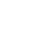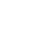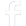#### Connect To Facebook#### WhatsApp Quick Chat#### Get Instant Call Back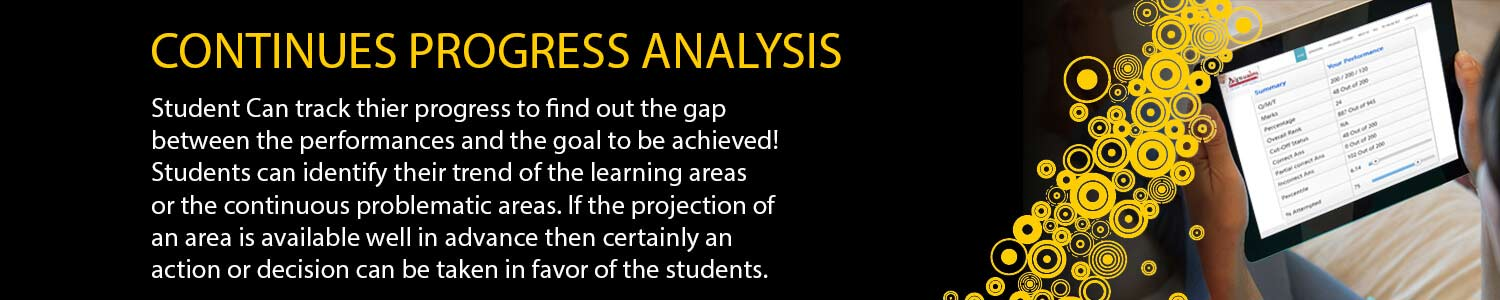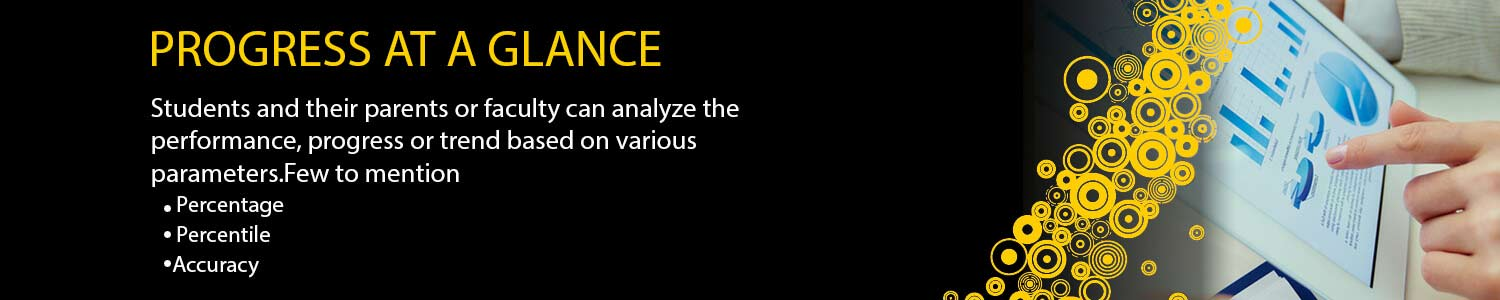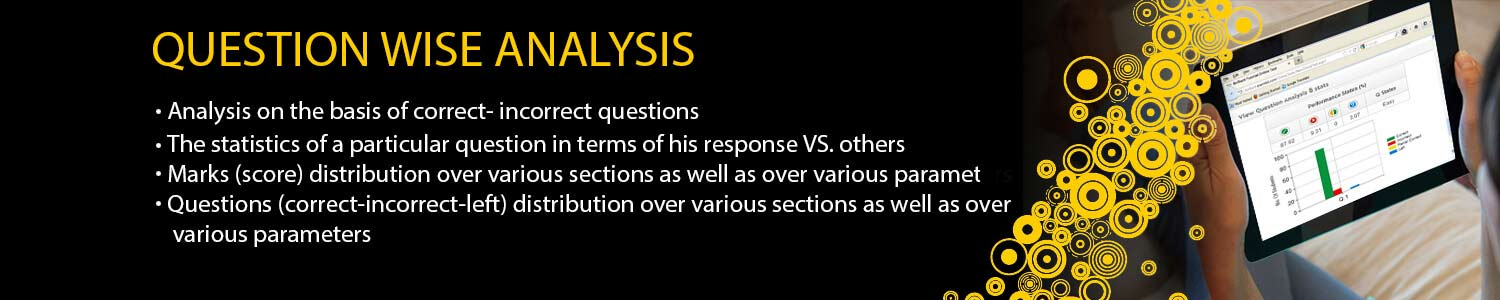## Offers## News & Events##### OTS (ONLINE TEST SERIES)
# DATE TEST TYPE MODULES IIT-JAM STATISTICSTEST SERIES 2019 4 Jan 19 MWT-01 Random Variable & Standard Distribution Random Variables Probability mass function, probability density function and cumulative distribution functions, distribution of a function of a random variable. Mathematical expectation, moments and moment generating function. Chebyshev's inequality. Standard Distributions Binomial, negative binomial, geometric, Poisson, hypergeometric, uniform, exponential, gamma, beta and normal distributions. Poisson and normal approximations of a binomial distribution. 7 Jan 19 MWT-02 Joint Distribution Joint Distributions : Joint, marginal and conditional distributions. Distribution of functions of random variables. Joint moment generating function. Product moments, correlation, simple linear regression. Independence of random variables. Sampling distributions: Chi-square, t and F distributions, and their properties. 9 Jan 19 MWT-03 Real Analysis II Probability: Axiomatic definition of probability and properties, conditional probability, multiplication rule. Theorem of total probability. Bayes' theorem and independence of events. Limit Theorems: Weak law of large numbers. Central limit theorem (i.i.d.with finite variance case only). 11 Jan 19 MWT-04 Estimation Unbiasedness, consistency and efficiency of estimators, method of moments and method of maximum likelihood. Sufficiency, factorization theorem. Completeness, Rao-Blackwell and Lehmann-Scheffe theorems, uniformly minimum variance unbiased estimators. Rao-Cramer inequality. Confidence intervals for the parameters of univariate normal, two independent normal, and one parameter exponential distributions. 12 Jan 19 MWT-05 Estimation Unbiasedness, consistency and efficiency of estimators, method of moments and method of maximum likelihood. Sufficiency, factorization theorem. Completeness, Rao-Blackwell and Lehmann-Scheffe theorems, uniformly minimum variance unbiased estimators. Rao-Cramer inequality. Confidence intervals for the parameters of univariate normal, two independent normal, and one parameter exponential distributions. 14 Jan 19 MWT-06 Testing of Hytotheses Basic concepts, applications of Neyman-Pearson Lemma for testing simple and composite hypotheses. Likelihood ratio tests for parameters of univariate normal distribution. 15 Jan 19 MWT-07 Testing of Hytotheses Basic concepts, applications of Neyman-Pearson Lemma for testing simple and composite hypotheses. Likelihood ratio tests for parameters of univariate normal distribution. 18 Jan 19 MWT-08 Mathematics Sequences and Series: Convergence of sequences of real numbers, Comparison, root and ratio tests for convergence of series of real numbers. Differential Calculus : Limits, continuity and differentiability of functions of one and two variables. Rolle's theorem, mean value theorems, Taylor's theorem, indeterminate forms, maxima and minima of functions of one and two variables. Integral Calculus: Fundamental theorems of integral calculus. Double and triple integrals, applications of definite integrals, arc lengths, areas and volumes. Matrices: Rank, inverse of a matrix. Systems of linear equations. Linear transformations, eigenvalues and eigenvectors. Cayley-Hamilton theorem, symmetric, skew-symmetric and orthogonal matrices. 21 Jan 19 FLT-01 Full Length Test As per Exam Pattern. 24 Jan 19 FLT-02 Full Length Test As per Exam Pattern. 27 Jan 19 FLT-03 Full Length Test As per Exam Pattern. 30 Jan 19 FLT-04 Full Length Test As per Exam Pattern.

## Offers## News & Events